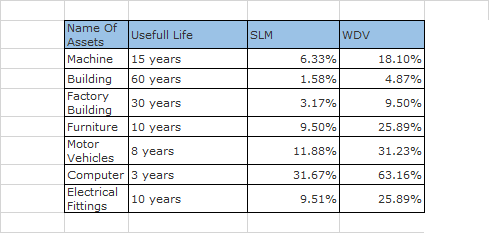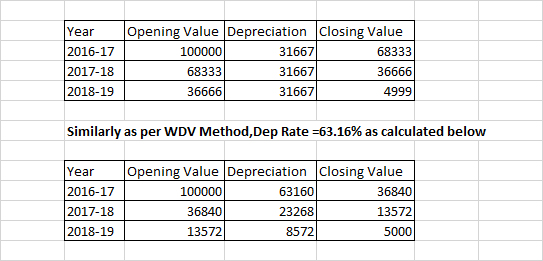Depreciation Chart

Accounts and Finance
Step 5 Preparing Projections and Budgets

It is used by Companies (Private Limited/Limited Companies) while making their books of accounts Hence,it is not of use of Proprietorship and Partnership

#### Rates Used in Companies Act

Both WDV and SLM Methods can be used Rate depends upon Useful Life of Asset In Companies Act Useful life is defined Rates are calculated assuming scrap value of 5%

For example

For Computer ,useful life is 10 years

#### Depreciation Rates on different assets#### How are Depreciation Rates Calculated

In Companies Act Useful life is defined Rates are calculated assuming scrap value of 5%

For example
For Computer ,useful life is 3 years

Suppose we purchase Computer for 100000 Scrap Value is 5%=5000 Depreciation Charged=100000-5000=95000 Depreciation Charged as per SLM Method is 95000/3=31666.67  Depreciation %=31.667%To learn how to calculate this,Learn Goal Seek Excel formula on teachoo

#### How is Depreciation Presented in Books?

Concept of Accumulated Depreciation is followed where Assets are shown at Gross Value Depreciation of different years are Added (Accumulated)

Example 1:-

SLM Method Computation and Presentation

Get live Maths 1-on-1 Classs - Class 6 to 12